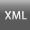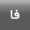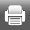Volume 29, Issue 4 (2015)geores 2015, 29(4): 229-240 Back to browse issues page
The analyze occurrences daily precipitation probability in Iran and forecast by using Markov chain model
Zahra mahavarpour *
Department Of Climateology ,Isfahan University,Isfahan,Iran , zarrin_mah2010@yahoo.com
Abstract:   (1517 Views)
In this paper occurrences of precipitation in Iran was analyzed according to random processes by the applying of markov chain model. In order to do that occurrence and non-occurrence of rainfall of asfezari data base for the period of 1961/3/21 to 2004/12/31 was used. This data are for 7187 pixel and 15991 days. By using markov chain model first order two state dry and wet frequency matrix was made. And then by the method of maximum likelihood transmission probability matrix was developed. By Chi-square goodness data following from chosen model was confirmed. By frequently powering this matrix stable probability and daily return period of rainy and non-rainy states was estimated. Period of daily returns and non-rainy days was estimated 7 and 1 respectively. So probability of precipitation was 0.1449 and probability of non-occurrence was estimated 0.8551. Return period of precipitation with lasting 1 to 3 days was calculated for all the months. The maximum likelihood of occurrence with precipitation was in March with the amount of 0.27. The shortest return period. The Shortest return period with lasting of 1 to 2 days was in February and March with the amount of 5.6 and 3.7 respectively.
Keywords: precipitation, Asfazari database, Markov chain, transition probability matrix.